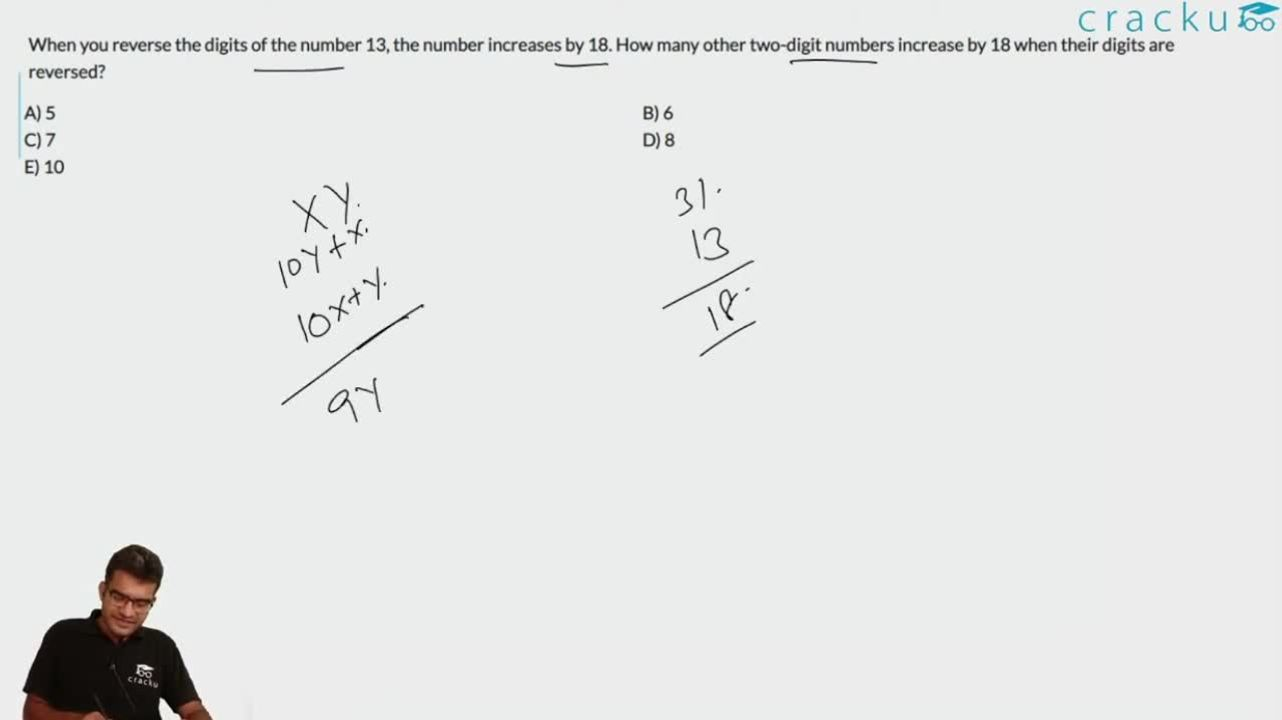Question 20

# When you reverse the digits of the number 13, the number increases by 18. How many other two-digit numbers increase by 18 when their digits are reversed?

Solution

Let the number be xy
10y + x = 10x + y + 18
=> 9y - 9x = 18
=> y - x = 2
So, y can take values from 9 to 4 (since 3 is already counted in 13)
Number of possible values = 6

### View Video Solution